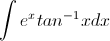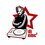Please solve this integral$\int \mathrm{e}^x tan^{-1}x\mathrm{d}x$Note by Krishna Jha
6 years, 3 months ago

This discussion board is a place to discuss our Daily Challenges and the math and science related to those challenges. Explanations are more than just a solution — they should explain the steps and thinking strategies that you used to obtain the solution. Comments should further the discussion of math and science.

When posting on Brilliant:

• Use the emojis to react to an explanation, whether you're congratulating a job well done , or just really confused .
• Ask specific questions about the challenge or the steps in somebody's explanation. Well-posed questions can add a lot to the discussion, but posting "I don't understand!" doesn't help anyone.
• Try to contribute something new to the discussion, whether it is an extension, generalization or other idea related to the challenge.
• Stay on topic — we're all here to learn more about math and science, not to hear about your favorite get-rich-quick scheme or current world events.

MarkdownAppears as
*italics* or _italics_ italics
**bold** or __bold__ bold

- bulleted
- list

• bulleted
• list

1. numbered
2. list

1. numbered
2. list
Note: you must add a full line of space before and after lists for them to show up correctly
paragraph 1

paragraph 2

paragraph 1

paragraph 2

> This is a quote
This is a quote
# I indented these lines
# 4 spaces, and now they show
# up as a code block.

print "hello world"
# I indented these lines
# 4 spaces, and now they show
# up as a code block.

print "hello world"
MathAppears as
Remember to wrap math in $$...$$ or $...$ to ensure proper formatting.
2 \times 3 $2 \times 3$
2^{34} $2^{34}$
a_{i-1} $a_{i-1}$
\frac{2}{3} $\frac{2}{3}$
\sqrt{2} $\sqrt{2}$
\sum_{i=1}^3 $\sum_{i=1}^3$
\sin \theta $\sin \theta$
\boxed{123} $\boxed{123}$

Sort by:

Here $tan^{-1}x$ is the inverse tangent function and can also be written as $arctan(x)$.

- 6 years, 3 months ago

Take z=arctan(x), then take dz =dx/(1+x^2)=dx/(1+tan^2z)=dx/(sec^2x)..................so dx=dz(sec^2z)...............so the integral reduces to [ integral e^(tan(z)) sec^2(z)) dz.....now substitute y=tan(z).....and tada u'll ger it!! Hope u understand it.........

(N.B..here sec^2(z) means sec squared z.................okay?)

- 6 years, 3 months ago

Man... You missed out a z....the integral is $\int\mathrm{e}^{tanz}z(sec^{2}z)\mathrm{d}z$.... coz there was an $arctan(x)$ there... u have to back substitute $arctan(x)=z$... Now please solve this further... :-P..

- 6 years, 3 months ago

- 6 years, 3 months ago

You should check this.

- 6 years, 3 months ago

Pls tell me how to solve this integral with the Ei(x) function..

- 6 years, 3 months ago

$\frac{1}{2} e^i i \text{Ei}(-i+x)-\frac{1}{2} i e^{-i} \text{Ei}(i+x)+e^x \tan ^{-1}(x)$

- 6 years, 3 months ago

HOW???

- 6 years, 3 months ago

Wolfram | Alpha

- 6 years, 3 months ago

Why not use integration by parts

- 6 years, 3 months ago

Use it and show me how do u do it...

- 6 years, 3 months ago